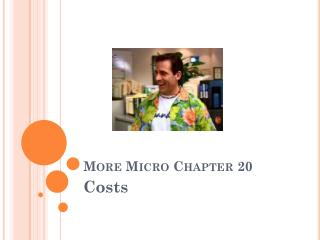Download PresentationMore Micro Chapter 20

# More Micro Chapter 20 - PowerPoint PPT PresentationDownload Presentation## More Micro Chapter 20

- - - - - - - - - - - - - - - - - - - - - - - - - - - E N D - - - - - - - - - - - - - - - - - - - - - - - - - - -
##### Presentation Transcript

1. Average Fixed cost (AFC) • Divide the total fixed cost by the output (Q) • AFC = TFC/Q • Since fixed costs are constant, AFC must decline as output increases • Referred to as “Spreading the Overhead”

2. Marginal Cost (MC) • The extra or additional cost of producing 1 more unit of output • MC = change in TC/change in Q

3. Average variable cost (AVC) • Divide total variable cost by output • TVC/Q • AVC graph is “u” shaped

4. Average total cost (ATC) • ATC = TC/Q • ATC = TFC/Q + TVC/Q • ATC = AFC + AVC

5. Long-Run Production Costs Long-Run ATC Curve ATC-1 ATC-5 ATC-2 Long-Run ATC ATC-4 ATC-3 Average Total Costs Output The Long-Run ATC Curve Just “Envelopes” the Short Run ATCs

6. G 20.1 \$200 150 100 Costs 50 0 10 1 2 3 4 5 6 7 8 9 Q Average and Marginal Costs • MC intersects Both ATC and AVC at their min MC AFC ATC AVC AVC AFC

7. How it Applies • When the amount (MC) added to total cost is less than the current average total cost, ATC will fall • When the marginal cost exceeds ATC, ATC will rise

8. Shifts of the Cost Curves • Changes in either resource prices or technology will cause costs to change and therefore the cost curves to shift • An increase in technology will shift the ATC curve downwards • An increase in resource prices would shift the curve upwards

9. Economies of Scale • Economies of Scale-Economies of mass production • Reduced per-unit cost as production increases • Reasons: labor and management specialization, efficient capital, per-unit advertising \$\$

10. Diseconomies of Scale • Increases in the average total cost of producing a product as the firm expands the size of its plant in the long run • ***delayed communication, top-heavy company, isolation of decision makers, shirking (avoiding work)

11. Constant Returns to Scale • Long run average costs do not change

12. Long-Run Production Costs Alternative Long-Run ATC Shapes Diseconomies Of Scale Constant Returns To Scale Economies Of Scale Average Total Costs Long-Run ATC q1 q2 Output Long-Run ATC Curve Where Economies Of Scale Exist

13. Sunk Costs • A cost that has already been incurred and can’t be recovered • Marginal Benefit v Marginal Cost • Assuming you can’t resell the good • Don’t cry over spilled milk